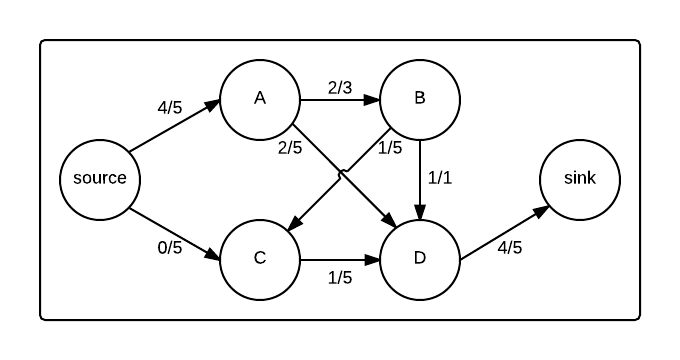# Find the augmenting path in the following graph

Which of the following paths is an augmenting path for the graph below?

The following graph shows a set of vertices and edges. Each edge shows two numbers: its current flow divided by its capacity.Graph in the middle of a max flow algorithm

×